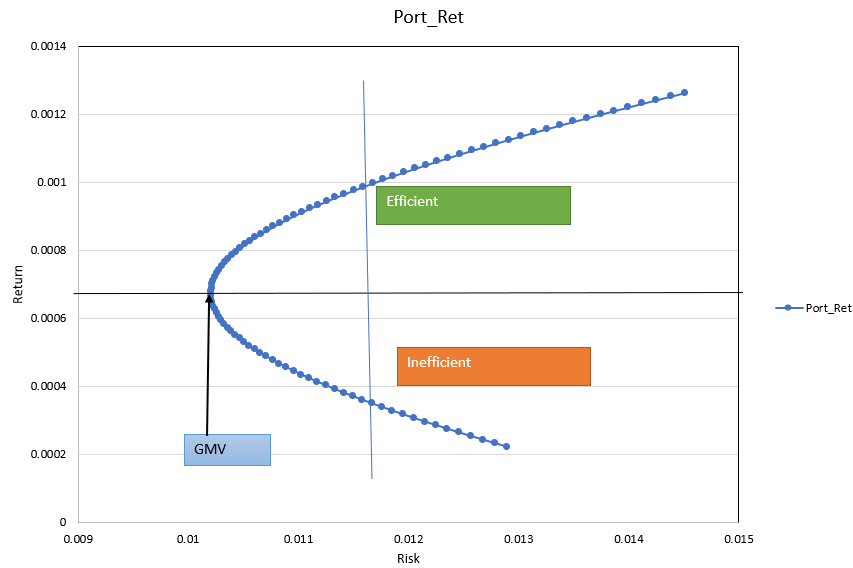## 12.3 Diversification & Markowitz Minimum Variance Portfolio

Chapter-4 from provides an easy to follow introduction and futher details.

Objective: Rational investors; maximise the expected return for a given risk, and they minimise the risk for a given return.

• A minimum variance set is the set of all portfolios that have the least volatility for each level of possible expected return.
• An efficient set (frontier) is the part of the minimum variance frontier that offers the highest expected return for each level of standard deviation.
• Given the level of risk or standard deviation, investors prefer positions with higher expected return and given the expected return, they prefer the positions of lower risk.
• Taking this into account, we can determine the minimum variance set.
• The point where the standard deviation is at its lowest is the global minimum variance portfolio (GMV).
• Portfolios that lie from the GMV portfolio upwards provide investors with the best risk–return combinations and thus are the candidates for the optimal portfolio.
• These portfolios are called the efficient set (frontier); and in order to be on the efficient frontier, the portfolios have to satisfy the following criterion:
• Given a particular level of standard deviation, the portfolios in the efficient set have the highest attainable expected rate of return.Figure 12.1: Efficient Frontier

### References

Szylar, Christian. 2013. Handbook of Market Risk. John Wiley & Sons.